Brownian motion, Ito's lemma, and the Black-Scholes formula (Part II)

Chuan Shi   2017-03-22 本文章706阅读

1

Recap

In the first article of this series, we explained the properties of the Brownian motion as well as why it is appropriate to use the geometric Brownian motion to model stock price movement. In addition, we gave the basic form of Ito's lemma, which paved the way for stochastic calculus and derivative pricing.

In today's article, we will begin with the general form of Ito's lemma and use it to solve the geometric Brownian motion, and derive the Black-Scholes differential equations and the Black-Scholes formula for European option pricing (this is also refered to as the Black-Scholes-Merton formula). To derive the BS formula, we will focus on a crucial idea in derivative pricing, the risk-neutral valuation. We will also put some effort to explain N(d_1) and N(d_2) which are two key elements in the BS formula. An understanding of them is essential to mastering the BS formula.

Before we step into technical details, let's have some fun first. The figure below shows Black, Merton, and Scholes. Scholes and Merton received the Nobel Memorial Prize in Economic Sciences in 1997 for their outstanding contributions in derivative pricing. The reason why Black was not awarded is that he passed away in 1995 and the Nobel prize is not poshumously awarded to scholars who died for more than 6 months.2

Ito's lemma

A Brownian motion with drift and diffusion satisfies the following stochastic differential equation (SDE), where μ and σ are some constants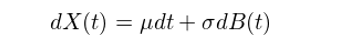More generally, the drift and diffusion coefficients can be functions of X(t) and t, rather than some constants. Let a(X(t),t) and b(X(t),t) be the drift and diffusion coefficients (in the example above, we therefore have a(X(t),t) = μ and b(X(t),t) = σ). We define a stochastic process that satisfies the following SDE the Ito dirft-diffusion process: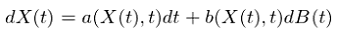Let f(X(t), t) be a continuous and smooth function of X(t) and t. With Ito's lemma, we derive: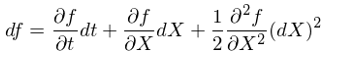Substitute dX with a(X(t),t)dt + b(X(t),t)dB in the equation above and ignore the higher order terms of dt. We finally derive the general form of Ito's lemma: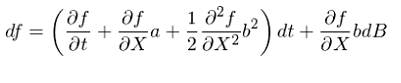From the SDE of f, it is easy to see that as a function of X and t, f itself is also a Ito drift-diffusion process. More importantly, comparing the expressions of dX and df, We see that both X and f are affected by the same underlying source of uncertainty, dB. In other words, the randomness possessed by the stochastic processes X and f comes from the same Brownian motion. This proves to be very important in the derivation of the BS formula.

We next apply Ito's lemma to solve the geometric Brownian motion.

3

Geometric Brownian motion

We model the price of some stock using the geometric Brownian motion. Let S denote the price of the stock, then it satisfies the following SDE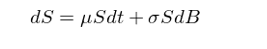where μ is the annual rate of return and σ is the standard deviation of that return. S is therefore a Ito process where a = μS and b = σS. To solve S, let f = lnS and apply Ito's lemma to df to derive the SDE of lnS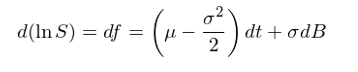The result shows that lnS is a Brownian motion with drift rate of μ – 0.5σ^2 and diffusion rate of σ. According to the property of the Brownian motion, within any interval [0, T], lnS (T) satisfies the following normal distribution: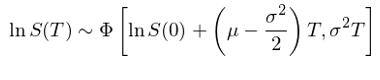A variable has a lognormal distribution if the natural logarithm of the variable is normally distributed. Therefore, when we model stock price using a geometric Brownian motion, the stock price satisfies a lognormal distribution.

Taking the integral of the SDE of lnS and then taking the exponential of the result, it is easy to derive how S varies with T: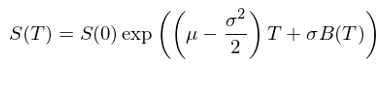This may seem counter-intuitive at the first glance. We assumed that the annual rate of return of the stock is μ. However, in the formula above, if we neglect the randomness from B(T) and only focus on the first part, it says that the stock price grows at a rate of μ – 0.5σ^2 rather than μ. What does this mean? What does the rate μ – 0.5σ^2 stand for?

The right answer is that μ – 0.5σ^2 is the continuously compounded rate of return per annumThis can be explained as follows. Let x be the continuously compounded rate per annum, and then S(T) follows S(T) = S(0)e^(xT) or x = (1/T)×(lnS(T) - lnS(0)). Since S is lognormally distributed, we know that lnS(T) – lnS(0) follows a normal distribution with mean (μ – 0.5σ^2)T and variance (σ^2)T. We can then solve for the x and it satisfies the following normal distribution: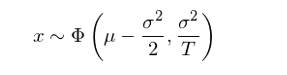While μ is the expected annual return, μ – 0.5σ^2 is the continuously compounded rate of return where it considers the variation σ. There difference is analog to the difference between the arithmetic mean and the geometric mean of return time series.

Consider the following example. Suppose that in the past 5 years a stock has returns of 15%, 20%, 30%, -20% and 25%. Their arithmetic mean is 14% and it means that the expected annual return μ is 14%. What is the continuously compounded rate of return? If we spent \$100 to purchase this stock 5 years ago and hold it since then, today our payback will be \$100 × 1.15 × 1.20 × 1.30 × 0.80 × 1.25 = \$179.4. This is equivalent to a 12.4% continuously compounded rate of return. It is lower than 14% due to the volatility of this return series.

4

Black-Scholes differential equation

In this section, we introduce the Black-Scholes differential equation. Note that the term 'stochastic' does not appear here, which means that the BS equation has no randomness, and this is great because no one likes uncertainty. You will know why it is the case after reading this section.

The assumptions used to derive the BS differential equation are as follows (Hull 2011):

1. European options are considered where they can be exercised only at maturity.

2. The stock price follows the geometric Brownian motion.

3. The short selling of securities with full use of proceeds is permitted.

4. There are no transactions costs or taxes. All securities are perfectly divisble.

5. There are no dividends during the life of the derivative.

6. During the life of the option, the standard deviation σ of the return of the underlying security is known and constant.

7. There are no riskless arbitrage opportunities.

9. The risk-free rate of interest, r, is constant and the same for all maturities.

It is true that some assumptions are not realistic. However, they are generally accepted for the purpose of pricing the options. Since the BS model was first proposed, academia has made great progress in derivative pricing. A number of improved models have been developed to correct various assumptions in the BS model. We next use the European call option as an example to introduce the BS differential equation.

Let C be the price of the call option. It is a function of S -- the price of the underlying stock -- and time t. Applying Ito's lemma to C(S, t) gives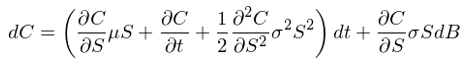Let's consider how S and C change within an infinitesimal time interval Δt. To do so, consider the discrete versions of dS and dC: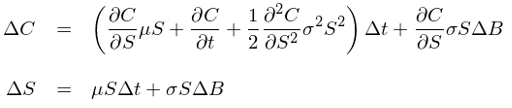We mentioned in section 2 of this article that the function f of a Ito process X is also a Ito process, and more importantly, the uncertainty of f and X comes from the same underlying Brownian motion. This means that the uncertainty in ΔC and ΔS comes from the same ΔB. It follows that a portfolio of the stock and this call option can be constructed so that this Brownian motion is eliminated. This portfolio is:The holder of this portfolio is short one derivative and long an amount ∂C/∂S of shares of the stock. It is easy to see that with such weights, the Brownian motion ΔB is perfectly eliminated in this portfolio. This technique of creating portfolio is called Delta hedging.

Let P be the value of the portfolio, then P's change within the interval Δt is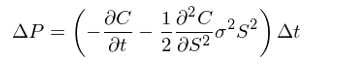Since ΔB does not appear in the expression of ΔP, it is only a function of time. By shorting one option and longing ∂C/∂S shares of stock, we have cancelled the risk within the time interval Δt and constructed a riskless portfolio. In a arbitrage-free market, this portfolio must earn the risk-free return of r, and therefore ΔP = rPΔt. Substituting ΔP and P = -C + (∂C/∂S)S into this equation gives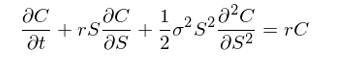This is the famous Black-Scholes differential equation. Since we removed the randomness by delta hedging, there is no stochastic term in this equation, and therefore it is a regular (partial) differential equation, rather than a stochastic differential equation. Solving this differential equation requires the correct boundary conditions. For European call option, its boundary condition is that at t = T (when the option matures), C must satisify C = max(S(T) - K, 0), where K is the strike price.

5

Risk-neutral valuation

It is true that we can derive the price of the call option by solving the differential equiation with appropriate boundary conditions. However, in derivative pricing, there is another very useful method that could not be emphasized too much. It is called risk-neutral valuation.

You may get confused and ask if that is the case why we put a lot of effort to derive the BS differential equation,

However, risk-neutral valuation comes from one key property of the BS differential equation:

"The BS differential equation does not involve any variables that are affected by the risk preferences of investors. The variables that do appear in the equation are the current stock price, time, stock price volatility, and the risk-free rate of interest. All are independent of risk preferences."

We see from the BS differential equation that the expectation return of the stock, μ, does not appear in it. Apparently, μ does depend on risk preferences of investors. The higher the level of risk aversion by investors, the higher μ will be for any given stock. It is fortunate that when we use delta hedging to remove the Brownian motion, μ also happens to drop out in the derivation. This is a happy accident.

Because the BS differential equation is independent of risk prefrences, it means it cannot impact its solution. Any set of risk preferences can be used when evaluating C.  In particular, the very simple assumption that all investors are risk neutral can be made.

To price any derivative, we must know two things:

1. Its expected price at its maturity. Since the price of a derivative is a function of the price of the underlying asset, it is therefore dependent of the expected return of the underlying asset, μ.

2. After we know its expected price at its maturity, we must compute its present value at t = 0. It means that we must know the discount rate for the derivative.

Unfortunately, in the real world, we could hardly know any of them. This is why the risk-neutral valuation is very useful, as described by Hull (2011):

"In a world where investors are risk neutral, the expected return on all investment assets is the risk-free rate of interest, r. The reason is that risk-neutral investos do not require a premium to induce them to take risks. It is also true that the present value of any cash flow in a risk-neutral world can be obtained by discounting its expected value at the risk-free rate. The assumption that the world is risk neutral does, therefore, considerably simplify the analysis of derivatives."

A derivative can be valued using risk-neutral valuation by using the following procedure (Hull 2011):

1. Assume that the expected return from the underlying asset is the risk-free interest rate, r (i.e., assume μ = r).

2. Calculate the expected payoff from the derivative.

3. Discount the expected payoff at the risk-free interest rate.

Hull (2011) provdes some great comments on the risk-neutral valuation: It is important to appreciate that risk-neutral valuation is merely an artificial device for obtaining solutions to the BS differential equation. The solutions that are obtained are valid in all worlds, not just those where investors are risk neutral. When we move from a risk neutral world to a risk-averse world, two things happen. The expected growth rate in the stock price changes and the discount rate that must be used for any payoffs from the derivative changes. It happens that these two changes always offset each other exactly.

We will next derive the price of European call option, C, using the risk-neutral valuation.

6

Black-Scholes Formula for option pricing

The expected value of an European call option at maturity is E[max(S(T) – K, 0)], where S(T) is the stock price at t, and K is the strike price. With risk-neutral valuation, we assume that the expected return of S is the risk-free rate r, and the discount rate for the call option is also r. At maturity (t = T), the price of the call option is therefore: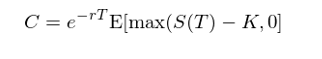Since S follows a lognormal distribution, it is not hard to compute E[max(S(T) – K, 0)] and doing that gives the famous Black-Scholes formula for option pricing. For call and put options, their prices C and P are: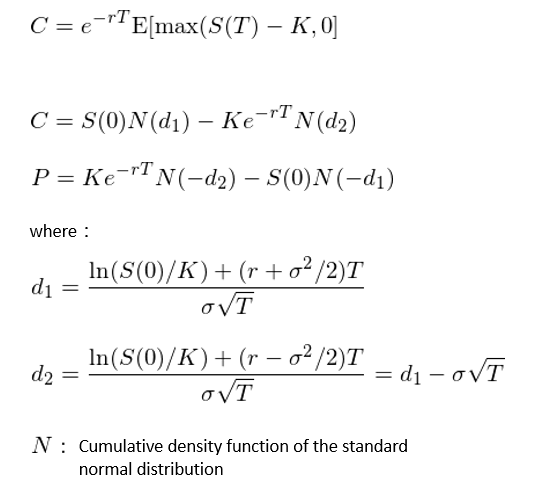With the help of a computer, we only need to provide values of the 5 inputs: current stock price S(0), strike price K, time to maturity T, risk-free interest rate r, and the standard deviation of the stock σ. By using these formulas, it will be very convenient to find the prices for both European call and put options.

But we must understand the meaning behind these formulas.

For any option, two uncertainty must be considered when pricing it:

1. Whether this option will be in-the-money at maturity. For example, if I purchase a call option, but the price of the underlying asset S is lower than the strike price K at T, then this option has no value.

2. If the option is in-the-money and we exercise it, how much our payoff will be. For example, with a call option, if the stock price is 110 and the strike price is 100, then by exercising, we earn 10 per share; if the stock price is 20, we earn 20 per share.

These two uncertainty corresponding to N(d_1) and N(d_2) in the BS formula.

We explain them using the call option. N in the BS formula is the cumulative density function of the standard normal distribution. Therefore, N(d_1) and N(d_2) represent two probabilities. In particular, N(d_2) is the probability that the option will be exercised in the risk-neutral world, i.e., it is prob(S(T) > K). It follows that the second term in C, i.e., Ke^(-rT)N(d_2) is the present value of the expected cost of exercising the option at maturity.

As for N(d_1), it is less intuitive than N(d_2). Let's put N(d_1) aside shortly and check the first term in C first. Since the second term of C is the expected cost, the first term of C must be the expected payoff (discounted to present) when the call option is exercised at T. Since we will only exercise the option when S(T) > K, then the expected value of this option conditioning on exercise is E[S(T) | S(T) > K]. Multipling this conditional expectation with the probability of exercise N(d_2) and then discounting it to today will give the first term of C. Then we have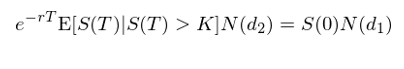Substituting S(0) with e^(-rT)E[S(T)] in the equation above derives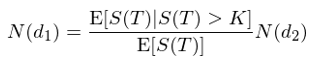Since E[S(T) | S(T) > K] > E[S(T)], we have N(d_1) > N(d_2). We then interpret N(d_1) as the probability of exercise weighted by the stock price in the risk-neutral world. This is because unlike the cost which is independent of S, the expected payoff is a function of S.

There is indeed other, yet more mathematical, interpretation about N(d_1). It is the probability of exercising the option in a risk-averse world where the market price of risk is measured by σ and the stock is used as the numeraire. It is perfectly fine to skip this interpretation as understanding it requires the knowledge of chagne of measure, equivalent martingale measure and change of numeraire. These concepts are beyond the scope of this article. Interested readers are recommended to take a look at Joshi (2008) for further discussion.

If we take the partial derivative of C against S, it turns out that N(d_1) happens to equal ∂C/∂S. In practice, some people therefore interpret N(d_1) as the measure of how sensitive of C to the change of S, although this interpretation is not quite correct.

Perhaps you will ask: the BS pricing formula only gives a theoretical price based on various strict assumptions, is it useful in the real world? Can people really build profitable strategies by utilizing the difference between the theoretical price and the actual price?

The key value of the BS pricing formula is that it provides a analytical tool. It allows us to calculate various risk exposures that the option experiences. This is very important for investors who want to use options (as well as other derivatives) in their portfolios. With the BS formula (i.e., taking partial derivatives), it is easy to calculate how derivative's price is impacted by the price of the underlying asset, time, interest rate, and the volatility. This gives the exposures of the derivative to these risk factors. There are two most popular risk factors, and they are: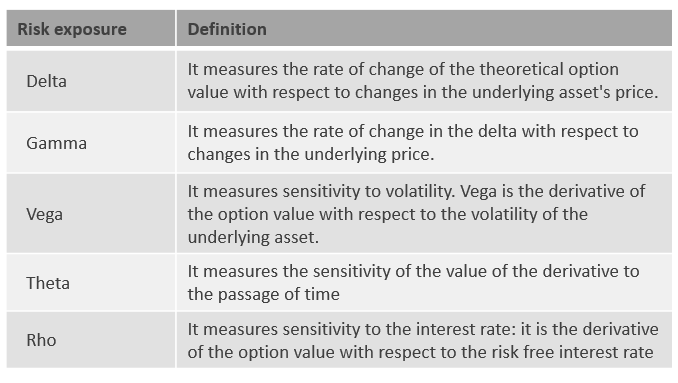They are also referred to Greeks since they are all Greek letters (note Vega is not a Greek letter). We will explain them in detail in another article in the future.

Another important usage of the BS formula is to calculate the implied volatility of the underlying asset. In the BS formula, all input parameters other than σ are very clear to all the investors, but people may take different values for σ. By using the option's price it is possible to inversely deduce the value of σ agreed by most of the investors (as it is calculated from the option's price) and this is called the implied volatility. The most famous example for implied volatility is the VIX. VIX is the ticker symbol for the Chicago Board Options Exchange (CBOE) Volatility Index, which shows the market's expectation of 30-day volatility. It is constructed using the implied volatilities of a wide range of S&P 500 index options.

7

Summary

This article starts with the general form of Ito's lemma. The derivation shows that a function of a Ito process is also a Ito process, and the randomness of the two processes is the same and comes from the same underlying Brownian motion. This fact is crucial to the derivation of the BS difference equation.

With the help of Ito's lemma, it is easy to solve the geometric Brownian motion, which is used to model stock price movement. Under this assumption, stock price follows a lognormal distribution and this is one of the assumptions used by the BS model.

Finally, by using delta hedging, we can construct a portfolio of the underlying stock and its derivative to eliminate the uncertainty and therefore derive the BS differential equation. With delta hedging, the risk preference of investors, μ, is also removed from the BS equation. This leads to a very important method to price the option, the risk-neutral valuation. It is applied to derive the prices for both the European call and put options and we also explain how to interpret these formulas. At the end of the article, we offer some discussion about how the BS formula is used in practice. It is used to measure the exposures of the derivative to various risk factors, and this can be of great value to investors who utilize options as an investment instrument.

References

Hull, J. C (2011). Options, Futures, and Other Derivatives (8th ed.). Prentice Hall.

Joshi, M. S. (2008). The Concepts and Practice of Mathematical Finance (Mathematics, Finance and Risk) (2nd ed.). Cambridge University Press.